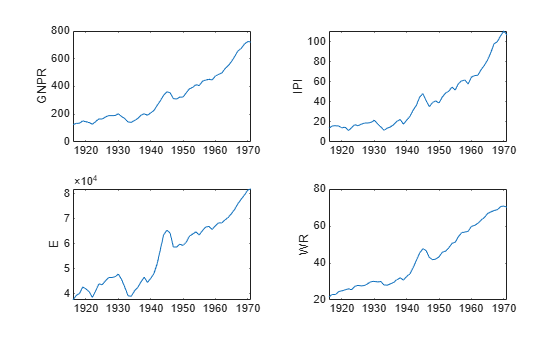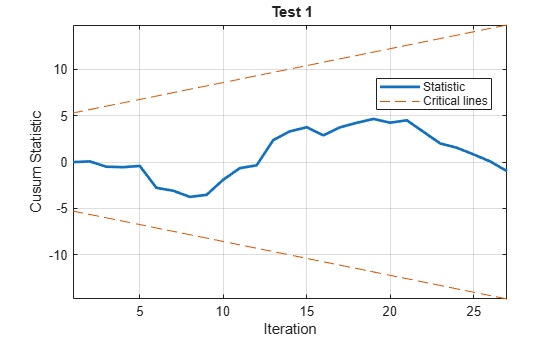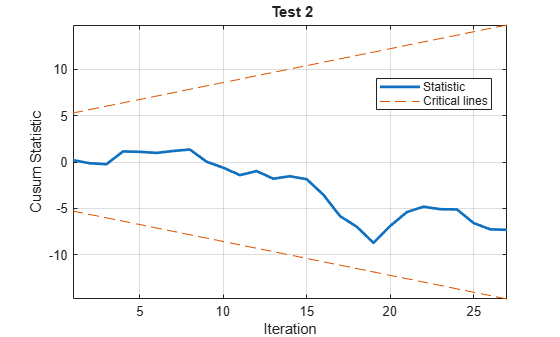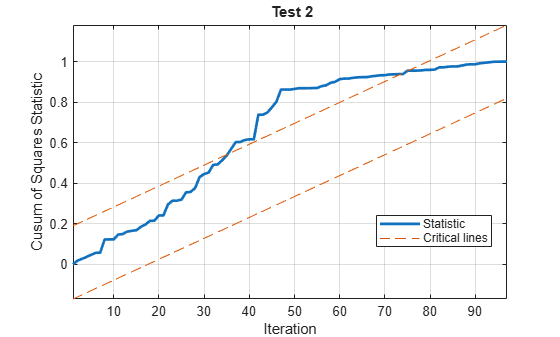# cusumtest

Cusum test for structural change

## Syntax

cusumtest(X,y)
cusumtest(Tbl)
cusumtest(___,Name,Value)
[h,H,Stat,W,B] = cusumtest(___)
cusumtest(ax,___)
[h,H,Stat,W,B,sumPlots] = cusumtest(___)

## Description

Cusum tests assess the stability of coefficients (β) in a multiple linear regression model of the form y = + ε. Inference is based on a sequence of sums, or sums of squares, of recursive residuals (standardized one-step-ahead forecast errors) computed iteratively from nested subsamples of the data. Under the null hypothesis of coefficient constancy, values of the sequence outside an expected range suggest structural change in the model over time.

example

cusumtest(X,y) plots both the sequence of cusums and the critical lines for conducting a cusum test on the multiple linear regression model y = Xβ + ε.

example

cusumtest(Tbl) plots using the data in the tabular array Tbl. The first numPreds columns are the predictors (X) and the last column is the response (y).

example

cusumtest(___,Name,Value) specifies options using one or more name-value pair arguments in addition to the input arguments in previous syntaxes. For example, you can specify which type of cusum test to conduct by using 'Test' or specify whether to include an intercept in the multiple regression model by using 'Intercept'.

example

[h,H,Stat,W,B] = cusumtest(___) returns: h, the test decisionH, the sequence of decisions for each iteration of the testStat, the sequence of test statisticsW, the sequence of recursive residualsB, the sequence of coefficient estimates 
cusumtest(ax,___) plots on the axes specified by ax instead of the current axes (gca). ax can precede any of the input argument combinations in the previous syntaxes.
[h,H,Stat,W,B,sumPlots] = cusumtest(___) additionally returns handles to plotted graphics objects. Use elements of sumPlots to modify properties of the plot after you create it.

## Examples

collapse all

Determine whether an explanatory model of real gross national product (GNP) is stable by plotting recursive residuals.

load Data_NelsonPlosser

The time series in the data set contain annual, macroeconomic measurements from 1860 to 1970. For more details, a list of variables, and descriptions, enter Description in the command line.

Several series have missing data. Focus the sample to measurements from 1915 to 1970.

span = (1915 <= dates) & (dates <= 1970);

Consider the multiple linear regression model

${\text{GNPR}}_{t}={\beta }_{0}+{\beta }_{1}{\text{IPI}}_{t}+{\beta }_{2}{\text{E}}_{t}+{\beta }_{3}{\text{WR}}_{t}.$

Collect the model variables into a tabular array. Position the response as the last variable.

Mdl = DataTable(span,[4,5,10,1]);

Plot the test statistics.

cusumtest(Mdl);The cusum series crosses the upper critical line after the 45th recursive regression, which indicates model instability.

Conduct cusum tests to assess whether there are structural changes in the equation for food demand around World War II. Implement forward and backward recursive regressions to obtain the test statistics.

Load the U.S. food consumption data set, which contains annual measurements from 1927 through 1962 with missing data due to the war.

load Data_Consumption

For more details on the data, enter Description at the command prompt.

Consider a model for consumption as determined by food prices and disposable income, and assess its stability through the economic shock through the war.

Plot the series.

P = Data(:,1); % Food price index I = Data(:,2); % Disposable income index Q = Data(:,3); % Food consumption index figure; plot(dates,[P I Q],'o-') axis tight grid on xlabel('Year') ylabel('Index') title('{\bf Time Series Plot of All Series}') legend({'Price','Income','Consumption'},'Location','SE')Measurements are missing from 1942 through 1947, which correspond to World War II.

To examine elasticities, apply the log transformation to each series.

LP = log(P); LI = log(I); LQ = log(Q);

Assume that log consumption is a linear function of the logs of food price and income. In other words,

${\text{LQ}}_{t}={\beta }_{0}+{\beta }_{1}{\text{LI}}_{t}+{\beta }_{2}\text{LP}+{\epsilon }_{t}.$

${\epsilon }_{t}$ is a Gaussian random variable with mean 0 and standard deviation ${\sigma }^{2}$.

Identify the indices before World War II. Plot log consumption with respect to the logs of food price and income.

preWarIdx = (dates <= 1941); figure scatter3(LP(preWarIdx),LI(preWarIdx),LQ(preWarIdx),[],'ro'); hold on scatter3(LP(~preWarIdx),LI(~preWarIdx),LQ(~preWarIdx),[],'b*'); legend({'Pre-war observations','Post-war observations'},... 'Location','Best') xlabel('Log price') ylabel('Log income') zlabel('Log consumption') title('{\bf Food Consumption Data}') % Get a better view h = gca; h.CameraPosition = [4.3 -12.2 5.3];Conduct forward and backward cusum tests using a 5% level of significance for each test. Plot the cusums.

cusumtest([LP,LI],LQ,'Direction',{'forward','backward'},'Plot','on');
RESULTS SUMMARY *************** Test 1 Test type: cusum Test direction: forward Intercept: yes Number of iterations: 27 Decision: Fail to reject coefficient stability Significance level: 0.0500*************** Test 2 Test type: cusum Test direction: backward Intercept: yes Number of iterations: 27 Decision: Fail to reject coefficient stability Significance level: 0.0500The plots and test results at the command line indicate that neither test rejects the null hypothesis that coefficients are stable.

Compare the results of the cusum tests with the results of a Chow test. Unlike cusum tests, Chow tests require a guess for the time point at which the structural break occurs. Specify that the break point is 1941.

bp = find(preWarIdx,1,'last'); chowtest([LP,LI],LQ,bp,'Display','summary');
RESULTS SUMMARY *************** Test 1 Sample size: 30 Breakpoint: 15 Test type: breakpoint Coefficients tested: All Statistic: 5.5400 Critical value: 3.0088 P value: 0.0049 Significance level: 0.0500 Decision: Reject coefficient stability 

The test results reject the null hypothesis that the coefficients are stable.

The Chow and cusum test results are not consistent. For details on cusum test limitations, see Limitations.

Check whether a cusum of squares test can detect a structural break in volatility in simulated data.

Simulate a series of data from this regression model

$\left\{\begin{array}{c}{y}_{t}=\left[\begin{array}{ccc}1& 2& 3\end{array}\right]{x}_{t}+{\epsilon }_{1t};\phantom{\rule{0.2777777777777778em}{0ex}}t=1,...,50\\ {y}_{t}=\left[\begin{array}{ccc}1& 2& 3\end{array}\right]{x}_{t}+{\epsilon }_{2t};t=51,...,100\end{array}.$

${x}_{t}$ is a series of observations from three standard Gaussian predictor variables. ${\epsilon }_{1t}$ and ${\epsilon }_{2t}$ are series of Gaussian innovations both with mean 0 and standard deviation 0.1 and 0.2, respectively.

rng(1); % For reproducibility T = 100; X = randn(T,3); sigma1 = 0.1; sigma2 = 0.2; e = [sigma1*randn(T/2,1); sigma2*randn(T/2,1)]; b = (1:3)'; y = X*b + e;

Conduct a cusum of squares test using a 5% level of significance. Plot the test statistics and critical region bands. Indicate that there is no model intercept. Request to return whether the test statistics cross into critical region at each iteration.

[~,H] = cusumtest(X,y,'Test','cusumsq','Plot','on',... 'Direction',{'forward','backward'},'Display','off','Intercept',false);Because the test statistics cross the critical lines at least once for both tests, the tests reject the null hypothesis of constant volatility at 5% level. The test statistics change direction around iteration 50, which is consistent with the simulated break in volatility in the data.

H is a 2-by-97 logical matrix containing the sequence of decisions for each iteration of each cusum of squares test. The first row corresponds to the forward cusum of squares test, and the second row corresponds to the backward cusum of squares test.

For the forward test, determine the iterations that result in the test statistics crossing the critical line.

bp = find(H(1,:) == 1)
bp = 1×35 24 25 26 27 28 29 30 31 32 33 34 35 36 37 38 39 40 41 42 43 44 45 46 47 48 49 50 51 52 53 54 55 56 57 58 

## Input Arguments

collapse all

Predictor data for the multiple linear regression model, specified as a numObs-by-numPreds numeric matrix.

numObs is the number of observations and numPreds is the number of predictor variables.

Data Types: double

Response data for the multiple linear regression model, specified as a numObs-by-1 numeric vector.

Data Types: double

Combined predictor and response data for the multiple linear regression model, specified as a numObs-by-numPreds + 1 tabular array.

The first numPreds columns of Tbl are the predictor data, and the last column is the response data.

Data Types: table

Axes on which to plot, specified as a vector of Axes objects with length numTests.

By default, cusumtest plots each test to a separate figure.

### Note

cusumtest removes observations with missing (NaN) values in the predictors or the response.

### Name-Value Pair Arguments

Specify optional comma-separated pairs of Name,Value arguments. Name is the argument name and Value is the corresponding value. Name must appear inside quotes. You can specify several name and value pair arguments in any order as Name1,Value1,...,NameN,ValueN.

Example: 'Intercept',false,'Test','cusumsq' indicates to exclude an intercept term from the regression model and to use the cumulative sum of squares statistic.

Indicate whether to include an intercept when cusumtest fits the regression model, specified as the comma-separated pair consisting of 'Intercept' and true, false, or a logical vector of length numTests.

ValueDescription
truecusumtest includes an intercept when fitting the regression model. numCoeffs = numPreds + 1.
falsecusumtest does not include an intercept when fitting the regression model. numCoeffs = numPreds.

Example: 'Intercept',false(3,1)

Data Types: logical

Type of cusum test, specified as the comma-separated pair consisting of 'Test' and 'cusum', 'cusumsq', or a string vector or cell vector of test-types of length numTests.

ValueDescription
'cusum'Cusum test statistic. See .
'cusumsq'Cusum of squares test statistic. See .

Example: 'Test','cusumsq'

Data Types: char | cell | string

Iteration direction, specified as the comma-separated pair consisting of 'Direction' and 'forward', 'backward', or a string vector or cell vector of directions.

ValueDescription
'forward'

cusumtest computes recursive residuals beginning with the first numCoeffs + 1 observations. Then, cusumtest adds one at a time until it reaches numObs observations.

'backward'

cusumtest reverses the order of the observations, and then follows the same steps as in 'forward'.

Example: 'Direction','backward'

Data Types: char | cell | string

Nominal significance levels for the tests, specified as the comma-separated pair consisting of 'Alpha' and a numeric scalar or numeric vector of length numTests.

• For cusum tests, all elements of Alpha must be in the interval (0,1).

• For cusum of squares tests, all elements of Alpha must be in the interval [0.01,0.20].

Example: 'Alpha',0.1

Data Types: double

Flag indicating whether to display test results in the command window, specified as the comma-separated pair consisting of 'Display' and 'off' or 'summary'.

ValueDescriptionDefault Value When
'off'No displaynumTests = 1
'summary'For each test, display test results to the command windownumTests > 1

Example: 'Display','off'

Data Types: char | string

Flag indicating whether to plot test results, specified as the comma-separated pair consisting of 'Plot' and 'on' or 'off'.

Depending on the value of Test, the plots show the sequence of cusums or cusums of squares together with critical lines determined by the value of Alpha.

ValueDescriptionDefault Value When
'off'cusumtest does not produce any plots.cusumtest returns any output argument
'on'cusumtest produces individual plots for each test.cusumtest does not return any output arguments

Example: 'Plot','off'

Data Types: char | string

### Note

cusumtest determines the number of tests, numTests, by the length of any vector parameter value. cusumtest expands scalar or character array parameter values to vectors of length numTests. Vector values must all have length numTests. If any parameter value is a row vector, so is output h. Array outputs retain their specified dimensions.

## Output Arguments

collapse all

Cusum test decisions, returned as a logical scalar or logical vector of length numTests.

Hypotheses are independent of the value of Test:

 H0: Coefficients in β are equal in all sequential subsamples. H1: Coefficients in β change during the period of the sample.
• h = 1 indicates rejection of H0 in favor of H1.

• h = 0 indicates failure to reject H0.

Sequence of decisions for each iteration of the cusum tests, returned as a numTests-by-(numObsnumPreds) logical matrix.

Rows correspond to separate cusum tests and columns correspond to iterations.

• For tests in which Direction is 'forward', column indices correspond to times numPreds + 1,...,numObs.

• For tests in which Direction is 'backward', column indices correspond to times numObs – (numPreds + 1),...,1.

Rows corresponding to tests in which Intercept is true contain one less iteration, and the value in the first column of H defaults to false.

For a particular test (row), if any test decision in the sequence is 1, then h is 1, that is, h = any(H,2). Otherwise, h is false.

Sequence of test statistics for each iteration of the cusum tests, returned as a numTests-by-(numObsnumPreds) numeric matrix.

Rows correspond to separate cusum tests and columns correspond to iterations.

Values in any row depend on the value of Test. Indexing corresponds to the indexing in H.

Rows corresponding to tests in which Intercept is true contain one less iteration, and the value in the first column of Stat defaults to NaN.

Sequence of standardized recursive residuals, returned as a numTests-by-(numObsnumPreds) numeric matrix.

Rows correspond to separate cusum tests and columns correspond to iterations.

Rows corresponding to tests in which Intercept is true contain one less iteration, and the value in the first column of W defaults to NaN.

Sequence of recursive regression coefficient estimates, returned as a (numPreds + 1)-by-(numObsnumPreds)-by-numTests numeric array.

• B(i,j,k) corresponds to coefficient i at iteration j for test k.

At iteration j of test k, cusumtest estimates the coefficients using

B(:,j,k) = X(1:numPreds+j,inRegression)\y(1:numPreds+j);
inRegression is a logical vector indicating the predictors in the regression at iteration j of test k.

• During forward iterations, initially constant predictors can cause multicollinearity. Therefore, cusumtest holds out constant predictors until their data change. For iterations in which cusumtest excludes predictors from the regression, corresponding coefficient estimates default to NaN. Similarly, for backward regression, cusumtest holds out terminally constant predictors. For more details, see .

• Tests in which:

• Intercept is true contain one less iteration, and all values in the first column of B default to NaN.

• Intercept is false contain one less coefficient, and the value in the first row, which corresponds to the intercept, defaults to NaN.

Handles to plotted graphics objects, returned as a 3-by-numTests graphics array. sumPlots contains unique plot identifiers, which you can use to query or modify properties of the plot.

## Limitations

Cusum tests have little power to detect structural changes:

• Late in the sample period

• When multiple changes produce cancellations in the cusums

collapse all

### Cusum Tests

Cusum tests provide useful diagnostics for various model misspecifications, including gradual structural change, multiple structural changes, missing predictors, and neglected nonlinearities. The tests, formulated in , are based on cumulative sums, or cusums, of residual resulting from recursive regressions.

## Tips

• The cusum of squares test:

• Is a “useful complement to the cusum test, particularly when the departure from constancy of the [recursive coefficients] is haphazard rather than systematic” 

• Has greater power for cases in which multiple shifts are likely to cancel

• Is often suggested for detecting structural breaks in volatility

• Alpha specifies the nominal significance levels for the tests. The actual size of a test depends on various assumptions and approximations that cusumtest uses to compute the critical lines. Plots of the recursive residuals are the best indicator of structural change. Brown, et al. suggest that the tests “should be regarded as yardsticks for the interpretation of data rather than leading to hard and fast decisions” .

• To produce basic diagnostic plots of the recursive coefficient estimates having the same scale for test n, enter

plot(B(:,:,n)')
recreg produces similar plots, optionally using robust standard error bands.

## Algorithms

• cusumtest handles initially constant predictor data using the method suggested in  . If a predictor's data is constant for the first numCoeffs observations and this results in multicollinearity with an intercept or another predictor, then cusumtest drops the predictor from regressions and the computation of recursive residuals until its data changes. Similarly, cusumtest temporarily holds out terminally constant predictors from backward regressions. Initially constant predictors in backward regressions, or terminally constant predictors in forward regressions, are not held out by cusumtest, and can lead to rank deficiency in terminal iterations.

• cusumtest computes critical lines for inference in essentially different ways for the two test statistics. For cusums, cusumtest solves the normal CDF equation in  dynamically for each value of Alpha. For the cusums of squares test, cusumtest interpolates parameter values from the table in , using the method suggested in . Sample sizes with degrees of freedom less than 4 are below tabulated values, and cusumtest cannot compute critical lines. Sample sizes with degrees of freedom greater than 202 are above tabulated values, and cusumtest uses the critical value associated with the largest tabulated sample size.

 Brown, R. L., J. Durbin, and J. M. Evans. “Techniques for Testing the Constancy of Regression Relationships Over Time.” Journal of the Royal Statistical Society, Series B. Vol. 37, 1975, pp. 149–192.

 Durbin, J. “Tests for Serial Correlation in Regression Analysis Based on the Periodogram of Least Squares Residuals.” Biometrika. Vol. 56, 1969, pp. 1–15.